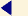back to menu
STRUCTURAL DESIGN - PART 17

English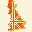27. Load combination with the seismic actions

The structural behaviour of the bridge under the seismic actions has been studied taking into account the non-linear behaviour of the masonry, as it was done for the flood force.

A very reliable seismic analysis can be performed using the step by step procedure, which is also well suited to the analysis of non-linear structures, because no superposition is invoked. A simplified modal response spectrum analysis has been performed; this type of analysis can be used strictly speaking only to structures whose response is not significantly affected by contributions from higher modes of vibration.

In the analysis the X-axis coincides with the longitudinal axis of the bridge, the Z-axis is in the transversal direction and the Y-axis is vertical.

For each direction the mode of vibration considered is the mode corresponding to the maximum participation factor.

Moreover the seismic actions of each direction have been applied contemporarily on the F.E. model with the combination factors according to Eurocode 8.

The following figures show the modes of vibrations used for the seismic analysis.

Mode of vibration along Z (mode of vibration no. 1)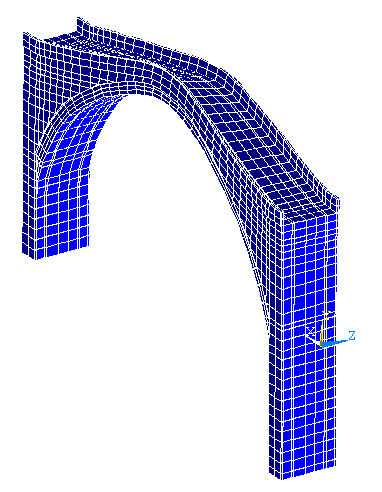Assonometric view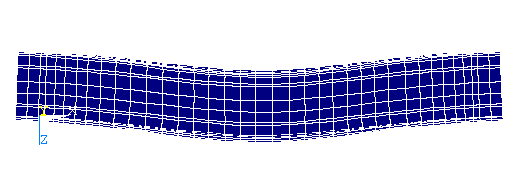Plan view

The first mode of vibration is translational, its eigenfrequency is 6.88 Hz and the participation mass is equal to 53 % of the total mass.

Mode of vibration along X (mode of vibration no. 2)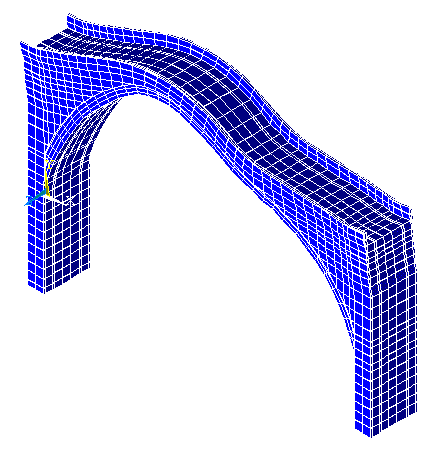Assonometric view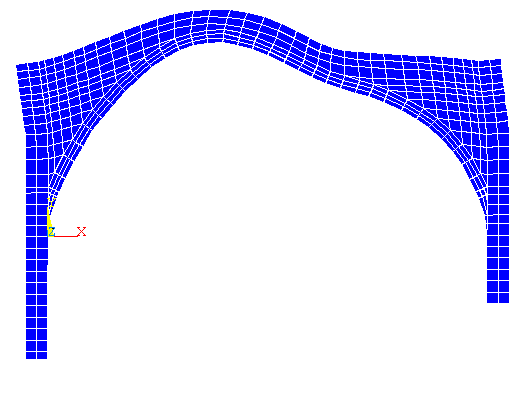Front view

The second eigenfrequency is equal to 10.48 Hz, the correspondent mode of vibration is anti-symmetrical in the vertical plane and the participation mass is equal to 25 % of the total mass.

Mode of vibration along Y (mode of vibration no. 4)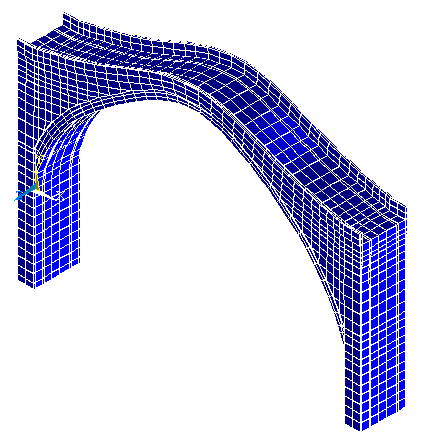Assonometric view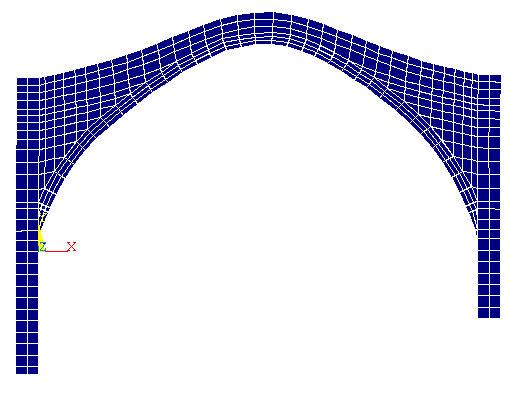Front view

The fourth eigenfrequency is 14.68 Hz and the correspondent participation mass is 37 % of the total mass.

The above shown modes of vibrations are uncoupled; the modal mass is high in one direction and small in the other two directions.

In the analysis under the seismic actions, in safety the total mass of the structure has been given to each of the above shown modes of vibration.

The effects of the earthquake have been determined applying statically equivalent loads Fi in each node, proportional to the nodal mass, in the direction parallel to the considered seismic action.

The forces have been determined with the following formula taken from Eurocode 8: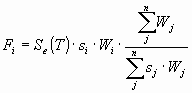.

where:

• Se(T) ordinate of the design spectrum,
• Wi , WJ weights of masses mi, mj,
• si , sJ displacements of masses mi, mj in the fundamental mode shape.

The weights have been computed with the following formula: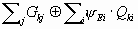,

where

• Gki characteristic value of the permanent loads,
• Qki characteristic value of the variable loads,
• y Ei reduction factors of the variable loads Qki.

Once the modes of vibration have been individualised, the response spectrum was defined.

The horizontal seismic action is described by two orthogonal components considered as independent and represented by the same response spectrum.

The vertical component of the seismic action is represented by the response spectrum as defined for the horizontal seismic action, but with the ordinates reduced as follows:

• for vibration periods T smaller than 0,15 s the ordinates are multiplied by factor of 0,7
• for vibration periods T greater than 0,50 s the ordinates are multiplied by a factor of 0,50
• for vibration periods T between 0,15 s and 0,50 s a linear interpolation is used.

The elastic response spectrum Se(T) normalised with respect to the ground acceleration a g is defined by the following expressions:

• for 0 Ł T Ł TB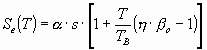• for TB Ł T Ł TC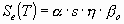• for TC Ł T Ł TD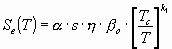• for TD Ł T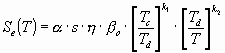where

• Se(T) ordinate of the elastic response spectrum,
• T the vibration period of the mode shape considered,
• a ratio between the peak acceleration of the ground and the gravity acceleration g (a = a g / g).

In particular the Stari bridge is located in a high seismic region; a gsh b o= 0.35 where:

• b o spectral acceleration amplification factor for 5% viscous damping,
• h damping correction factor with reference value h = 1 for 5% viscous damping for the masonry the Eurocode gives a viscous damping x = 8%, which implies h = 0.84,
• TB and TC limits of the constant spectral acceleration branch,
• TD value defining the beginning of the constant displacement range of the spectrum,
• k1 and k2 exponents which influence the shape of the spectrum for a vibration period greater than TC, TD respectively,
• s soil parameter.

N.B. The behaviour factor q is not used, because the performed analysis is non-linear.

The other coefficients are defined on the basis of the seismic-geological characteristics of the ground.

The bridge is located in a region classifiable as class A:

 Class S b o k1 K2 TB (sec) TC (sec) TD (sec) A 1 2.5 1.0 2.0 0.10 0.40 3.0 B 1 2.5 1.0 2.0 0.15 0.60 3.0 C 0.9 2.5 1.0 2.0 0.20 0.80 3.0

The final design spectrum is the following: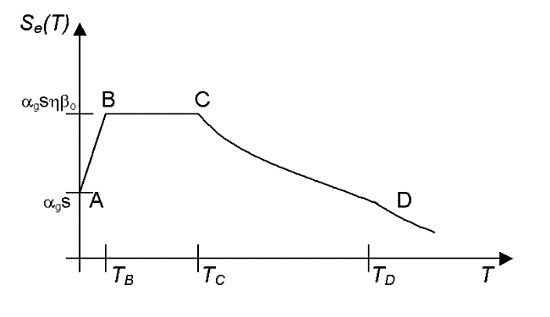where T is the vibration period of the mode shape considered and the characteristic points of the diagram are:

• A with Se(T)= 0.17 and TA=0 sec,
• B with Se(T)= 0.35 and TB=0.10 sec,
• C with Se(T)= 0.35 and TC=0.40 sec,

The spectral ordinates, relative to each direction, are:

• Z direction:

T = 0.15 sec Ţ Se(T) = 0.35,

• X direction:

T = 0.10 sec Ţ Se(T) = 0.35,

• Y direction:

T = 0.07 sec Ţ Se(T)= 0.30 * 70% = 0.21.

The horizontal and vertical seismic forces to be applied in each node of the structure have been calculated using the code "Matlab", elaborating some output files of the code "Ansys".

The expression of the seismic forces is: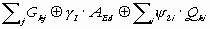.

Combination of the seismic forces

The seismic actions along the three directions X, Y and Z have been combined together, as prescribed by the Eurocode (see the following table).

 xxx Along X Along Y Along Z Prevailing earthquake along X 1.00 0.30 0.30 Prevailing earthquake along Y 0.30 1.00 0.30 Prevailing earthquake along Z 0.30 0.30 1.00

The seismic actions have been combined with the other actions according to Eurocode 8:where:

• Gki characteristic value of the permanent loads,
• g I importance factor of the structure,
• AEd design value of the seismic forces,
• Qki characteristic value of the variable loads,
• y 2i reduction factors of the variable loads (all zero)

CREDITS:

Intellectual property of this report and of the design drawings is owned by the University of Florence - Department of Civil Engineering

author of the text: Prof.Eng. Andrea Vignoli – other contributes have been mentioned in related paragraphs

© - General Engineering Workgroup -

SOURCE:

Final Design Reportback to menu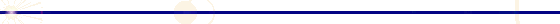GENERAL ENGINEERING - P.zzale Donatello 4 - 50132 Firenze - Italy - ph. +39 055 2345256 - fax. +39 055 2476074